# 谷歌面试题解析：单位换算## 问题描述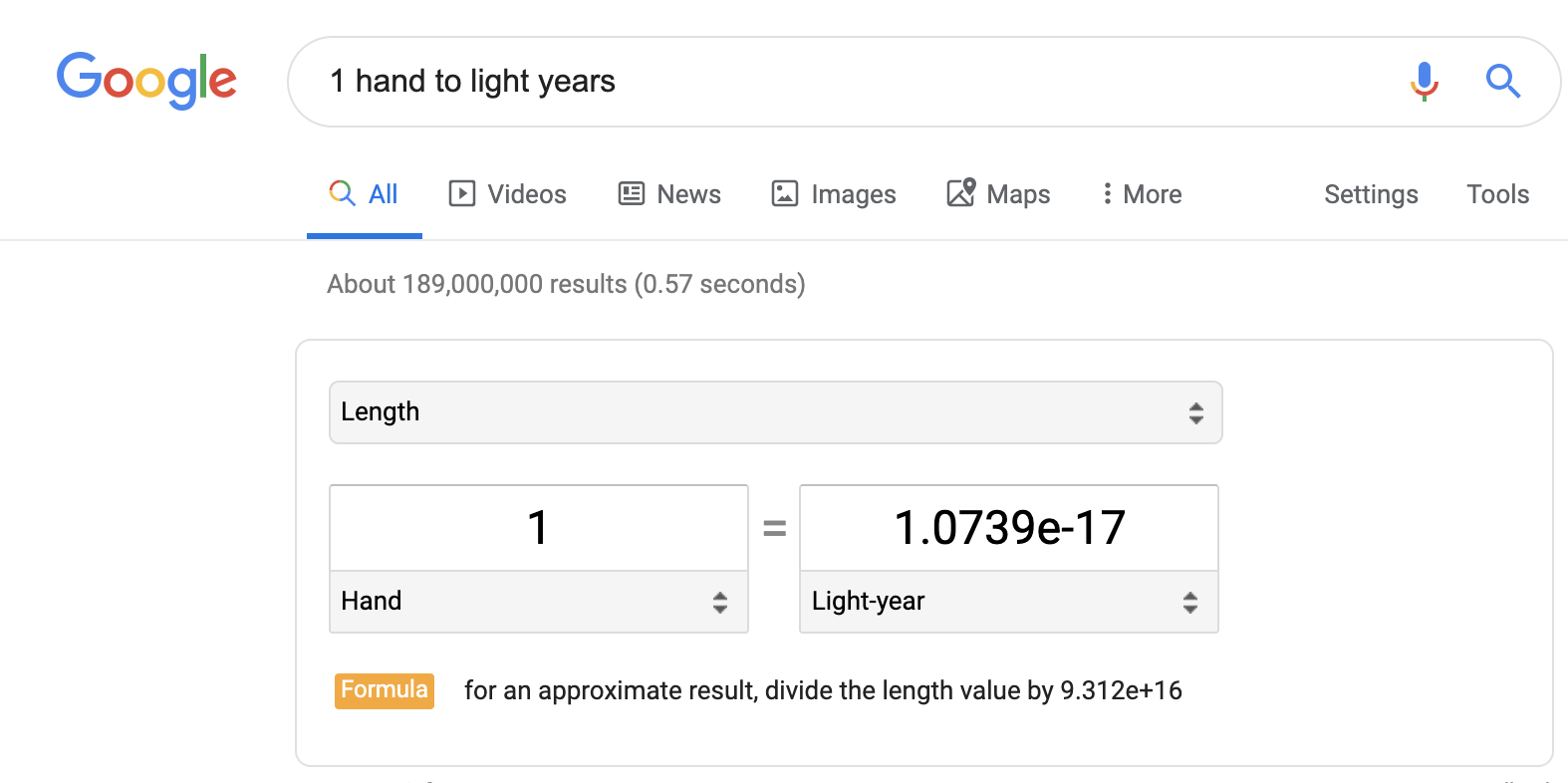• 一手等于 4 英寸；

• 4 英寸等于 0.33333 英尺；

• 0.33333 英尺等于 6.3125e-5 英里；

• 6.3125e-5 英里等于 1.0737e-17 光年。

……

## 第零步：直觉

• 一手等于 4 英寸；

• 4 英寸等于 0.33333 英尺；

• 0.33333 英尺等于 6.3125e-5 英里；

• 6.3125e-5 英里等于 1.0737e-17 光年。

## 第一步：直观的解决方案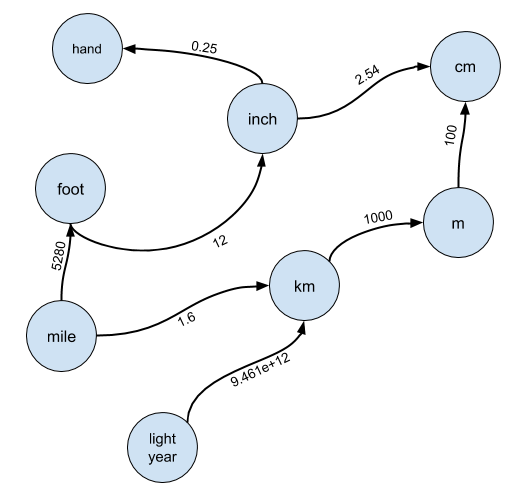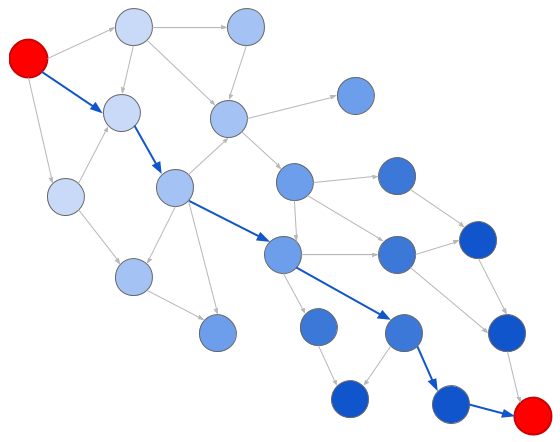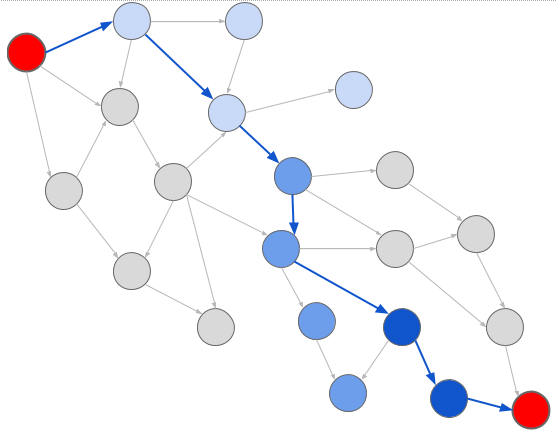class RateGraph(object):    def __init__(self, rates):        'Initialize the graph from an iterable of (start, end, rate) tuples.'        self.graph = {}        for orig, dest, rate in rates:            self.add_conversion(orig, dest, rate)     def add_conversion(self, orig, dest, rate):        'Insert a conversion into the graph.'        if orig not in self.graph:            self.graph[orig] = {}        self.graph[orig][dest] = rate     def get_neighbors(self, node):        'Returns an iterable of the nodes neighboring the given node.'        if node not in self.graph:            return None        return self.graph[node].items()            def get_nodes(self):        'Returns an iterable of all the nodes in the graph.'        return self.graph.keys()

from collections import deque def __dfs_helper(rate_graph, node, end, rate_from_origin, visited):    if node == end:        return rate_from_origin     visited.add(node){1}    for unit, rate in rate_graph.get_neighbors(node):        if unit not in visited:            rate = __dfs_helper(rate_graph, unit, end, rate_from_origin * rate, visited)            if rate is not None:                return rate    return None def dfs(rate_graph, node, end):    return __dfs_helper(rate_graph, node, end, 1.0, set())

DFS 是一种很好的搜索算法，如果存在解，它一定会把它找出来，但它缺少一个关键的属性：它不一定能找到最短路径。这跟我们很有关系，因为较短的路径意味着较少的跳数，较少的跳数意味着更少的浮点数乘法。为了解决这个问题，我们需要使用 BFS。

## 第二步：广度优先搜索

from collections import deque def bfs(rate_graph, start, end):    to_visit = deque()    to_visit.appendleft( (start, 1.0) )    visited = set()     while to_visit:        node, rate_from_origin = to_visit.pop()        if node == end:            return rate_from_origin        visited.add(node)        for unit, rate in rate_graph.get_neighbors(node):            if unit not in visited:                to_visit.appendleft((unit, rate_from_origin * rate))     return None

def add_conversion(self, orig, dest, rate):    'Insert a conversion into the graph. Note we insert its inverse also.'    if orig not in self.graph:        self.graph[orig] = {}    self.graph[orig][dest] = rate     if dest not in self.graph:        self.graph[dest] = {}    self.graph[dest][orig] = 1.0 / rate

## 第三步：计算

• 理解问题；

• 想出图数据结构；

• 将单位转换映射成图的路径；

• 知道可以使用图搜索算法来找到路径；

• 选择他们最喜欢的算法，并修改算法以便获得转换比率；

• 如果他们采用了 DFS 解决方案，也要知道它的缺点；

• 实现 BFS；

• 后退一步，检查边缘情况：

• 如果一个节点不存在该怎么办？

• 如果转换比率不存在呢？

• 需要考虑实现反向转换。

## 第五步：常量时间

“缓存”解决方案实际上已经离我们的目标不远了。我们得到了每个节点和其他节点之间的边，但我们真的需要保存从每个节点到其他节点的转换比率吗？如果我们只保存从一个节点到另一个节点的转换比率呢？

from collections import deque def bfs(rate_graph, start, end):    to_visit = deque()    to_visit.appendleft( (start, 1.0) )    visited = set()     while to_visit:        node, rate_from_origin = to_visit.pop()        if node == end:            return rate_from_origin        visited.add(node)        for unit, rate in rate_graph.get_neighbors(node):            if unit not in visited:                to_visit.appendleft((unit, rate_from_origin * rate))     return None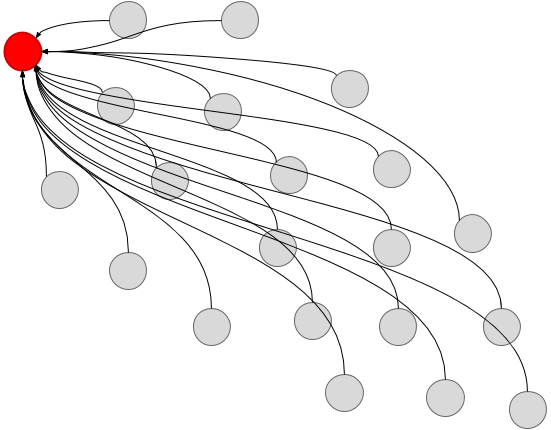from collections import deque def make_conversions(graph):    def conversions_bfs(rate_graph, start, conversions):        to_visit = deque()        to_visit.appendleft( (start, 1.0) )         while to_visit:            node, rate_from_origin = to_visit.pop()            conversions[node] = (start, rate_from_origin)            for unit, rate in rate_graph.get_neighbors(node):                if unit not in conversions:                    to_visit.append((unit, rate_from_origin * rate))         return conversions     conversions = {}    for node in graph.get_nodes():        if node not in conversions:            conversions_bfs(graph, node, conversions)    return conversions

def convert(conversions, start, end):    'Given a conversion structure, performs a constant-time conversion'    try:        start_root, start_rate = conversions[start]        end_root, end_rate = conversions[end]    except KeyError:        return None     if start_root != end_root:        return None     return end_rate / start_rate